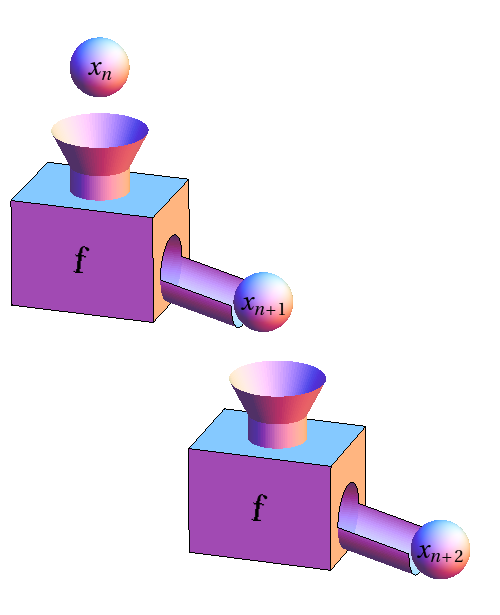# Math Insight

### Image: Function machine two iterationsThe composition of two function machines illustrates two iterations of a function. Here $x_{n+1}=f(x_n)$ and $x_{n+2}=f(x_{n+1})=f(f(x_{n}))$.

Image file: function_machine_two_iterations.png